# An Introduction to corrplot Package

## Introduction

The corrplot package is a graphical display of a correlation matrix, confidence interval. It also contains some algorithms to do matrix reordering. In addition, corrplot is good at details, including choosing color, text labels, color labels, layout, etc.

## Visualization methods

There are seven visualization methods (parameter method) in corrplot package, named "circle", "square", "ellipse", "number", "shade", "color", "pie".

Positive correlations are displayed in blue and negative correlations in red color. Color intensity and the size of the circle are proportional to the correlation coefficients.

library(corrplot)

## corrplot 0.84 loaded

M <- cor(mtcars)
corrplot(M, method = "circle")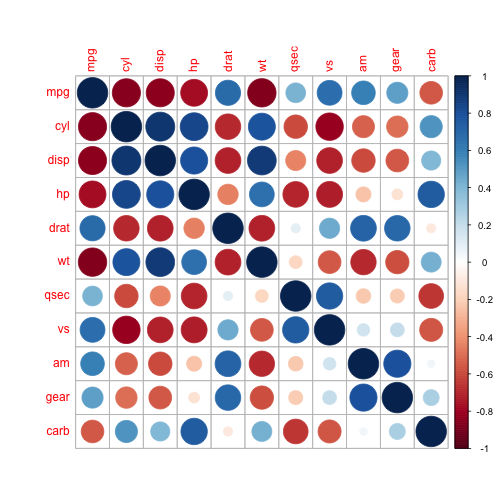corrplot(M, method = "square")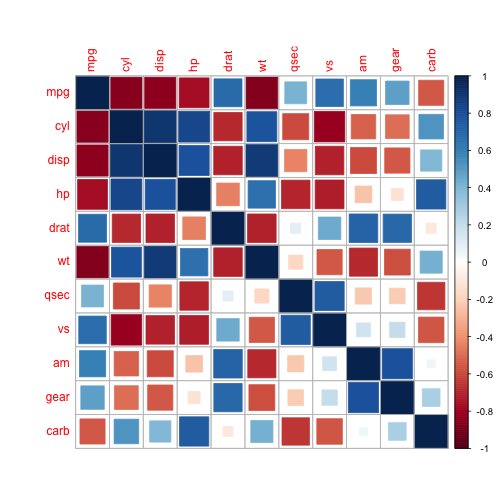corrplot(M, method = "ellipse")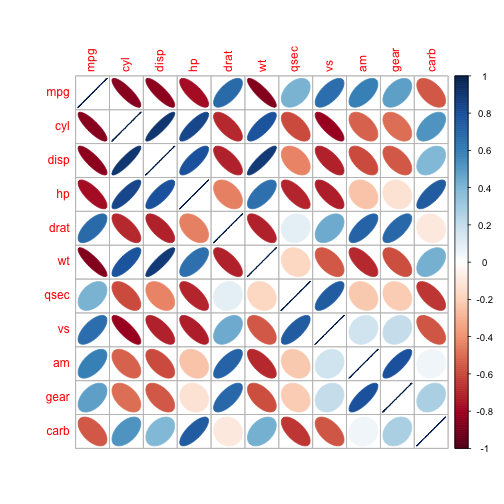corrplot(M, method = "number") # Display the correlation coefficient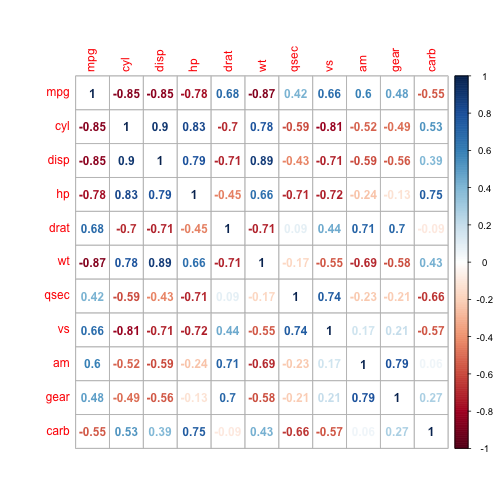corrplot(M, method = "shade")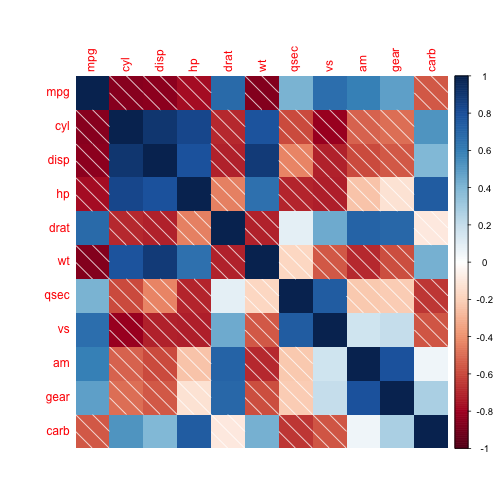corrplot(M, method = "color")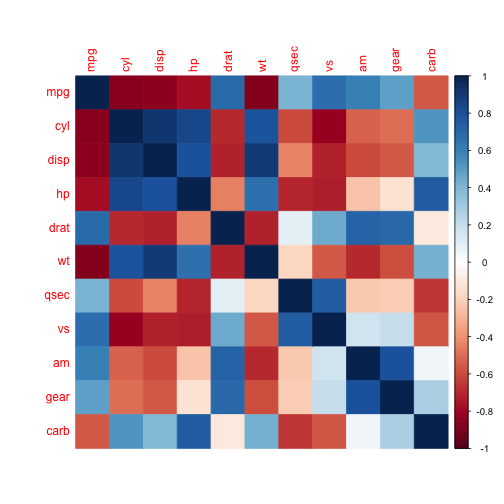corrplot(M, method = "pie")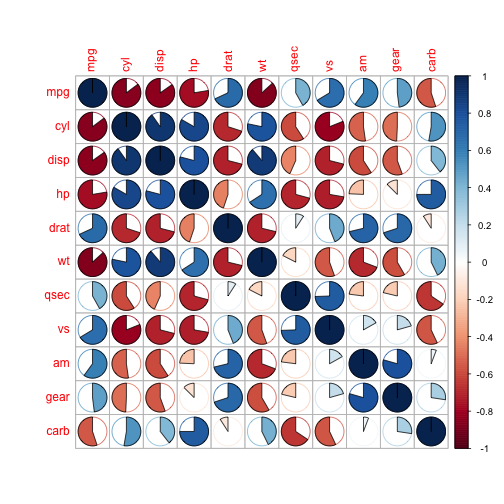## Layout

There are three layout types (parameter type):

• "full" (default) : display full correlation matrix
• "upper" : display upper triangular of the correlation matrix
• "lower" : display lower triangular of the correlation matrix
corrplot(M, type = "upper")
corrplot(M, type = "upper")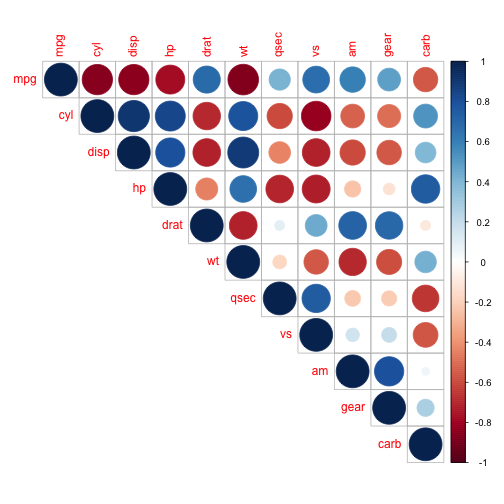corrplot.mixed() is a wrapped function for mixed visualization style.

corrplot.mixed(M)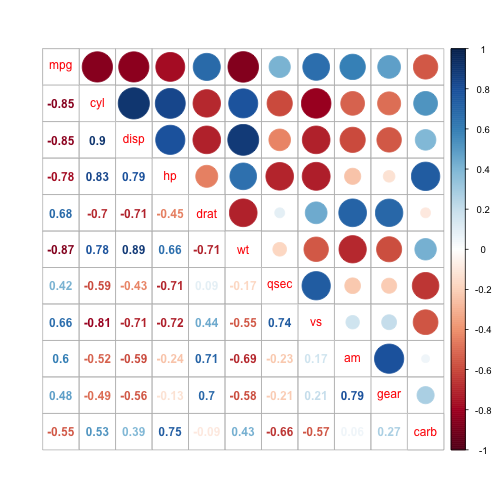corrplot.mixed(M, lower.col = "black", number.cex = .7)